# 8.7 Parametric equations: graphs  (Page 3/4)

 Page 3 / 4

Access the following online resource for additional instruction and practice with graphs of parametric equations.

## Key concepts

• When there is a third variable, a third parameter on which $\text{\hspace{0.17em}}x\text{\hspace{0.17em}}$ and $\text{\hspace{0.17em}}y\text{\hspace{0.17em}}$ depend, parametric equations can be used.
• To graph parametric equations by plotting points, make a table with three columns labeled $\text{\hspace{0.17em}}t,x\left(t\right),\text{\hspace{0.17em}}$ and $\text{\hspace{0.17em}}y\left(t\right).\text{\hspace{0.17em}}$ Choose values for $\text{\hspace{0.17em}}t\text{\hspace{0.17em}}$ in increasing order. Plot the last two columns for $\text{\hspace{0.17em}}x\text{\hspace{0.17em}}$ and $\text{\hspace{0.17em}}y.\text{\hspace{0.17em}}$ See [link] and [link] .
• When graphing a parametric curve by plotting points, note the associated t -values and show arrows on the graph indicating the orientation of the curve. See [link] and [link] .
• Parametric equations allow the direction or the orientation of the curve to be shown on the graph. Equations that are not functions can be graphed and used in many applications involving motion. See [link] .
• Projectile motion depends on two parametric equations: $\text{\hspace{0.17em}}x=\left({v}_{0}\mathrm{cos}\text{\hspace{0.17em}}\theta \right)t\text{\hspace{0.17em}}$ and $\text{\hspace{0.17em}}y=-16{t}^{2}+\left({v}_{0}\mathrm{sin}\text{\hspace{0.17em}}\theta \right)t+h.\text{\hspace{0.17em}}$ Initial velocity is symbolized as $\text{\hspace{0.17em}}{v}_{0}.\text{\hspace{0.17em}}\theta$ represents the initial angle of the object when thrown, and $\text{\hspace{0.17em}}h\text{\hspace{0.17em}}$ represents the height at which the object is propelled.

## Verbal

What are two methods used to graph parametric equations?

plotting points with the orientation arrow and a graphing calculator

What is one difference in point-plotting parametric equations compared to Cartesian equations?

Why are some graphs drawn with arrows?

The arrows show the orientation, the direction of motion according to increasing values of $\text{\hspace{0.17em}}t.$

Name a few common types of graphs of parametric equations.

Why are parametric graphs important in understanding projectile motion?

The parametric equations show the different vertical and horizontal motions over time.

## Graphical

For the following exercises, graph each set of parametric equations by making a table of values. Include the orientation on the graph.

$\left\{\begin{array}{l}x\left(t\right)=t\hfill \\ y\left(t\right)={t}^{2}-1\hfill \end{array}$

 $t$ $x$ $y$ $-3$ $-2$ $-1$ $0$ $1$ $2$ $3$

$\left\{\begin{array}{l}x\left(t\right)=t-1\hfill \\ y\left(t\right)={t}^{2}\hfill \end{array}$

 $t$ $-3$ $-2$ $-1$ $0$ $1$ $2$ $x$ $y$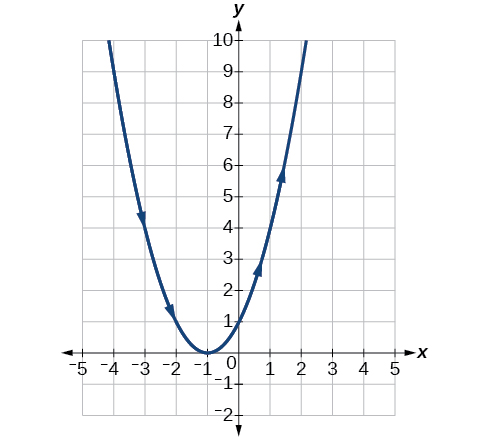$\left\{\begin{array}{l}x\left(t\right)=2+t\hfill \\ y\left(t\right)=3-2t\hfill \end{array}$

 $t$ $-2$ $-1$ $0$ $1$ $2$ $3$ $x$ $y$

$\left\{\begin{array}{l}x\left(t\right)=-2-2t\hfill \\ y\left(t\right)=3+t\hfill \end{array}$

 $t$ $-3$ $-2$ $-1$ $0$ $1$ $x$ $y$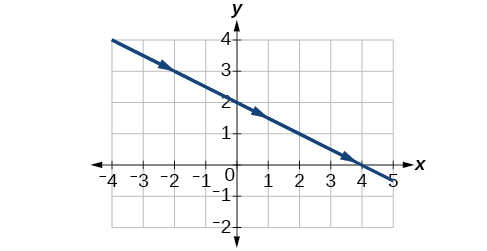$\left\{\begin{array}{l}x\left(t\right)={t}^{3}\hfill \\ y\left(t\right)=t+2\hfill \end{array}$

 $t$ $-2$ $-1$ $0$ $1$ $2$ $x$ $y$

$\left\{\begin{array}{l}x\left(t\right)={t}^{2}\hfill \\ y\left(t\right)=t+3\hfill \end{array}$

 $t$ $-2$ $-1$ $0$ $1$ $2$ $x$ $y$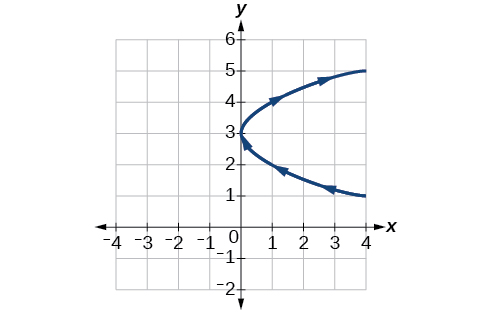For the following exercises, sketch the curve and include the orientation.

$\left\{\begin{array}{l}x\left(t\right)=t\\ y\left(t\right)=\sqrt{t}\end{array}$

$\left\{\begin{array}{l}x\left(t\right)=-\text{\hspace{0.17em}}\sqrt{t}\\ y\left(t\right)=t\end{array}$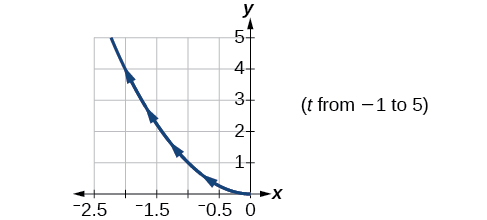$\left\{\begin{array}{l}x\left(t\right)=5-|t|\\ y\left(t\right)=t+2\end{array}$

$\left\{\begin{array}{l}x\left(t\right)=-t+2\\ y\left(t\right)=5-|t|\end{array}$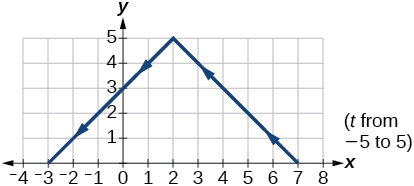$\left\{\begin{array}{l}x\left(t\right)=4\text{sin}\text{\hspace{0.17em}}t\hfill \\ y\left(t\right)=2\mathrm{cos}\text{\hspace{0.17em}}t\hfill \end{array}$

$\left\{\begin{array}{l}x\left(t\right)=2\text{sin}\text{\hspace{0.17em}}t\hfill \\ y\left(t\right)=4\text{cos}\text{\hspace{0.17em}}t\hfill \end{array}$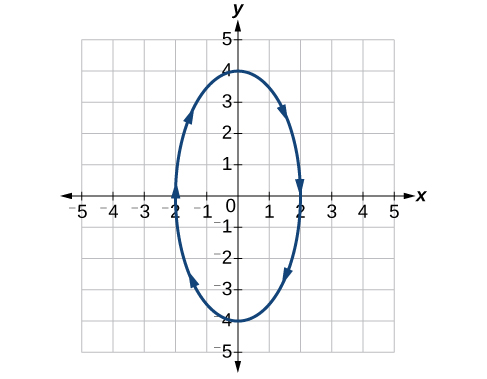$\left\{\begin{array}{l}x\left(t\right)=3{\mathrm{cos}}^{2}t\\ y\left(t\right)=-3\mathrm{sin}\text{\hspace{0.17em}}t\end{array}$

$\left\{\begin{array}{l}x\left(t\right)=3{\mathrm{cos}}^{2}t\\ y\left(t\right)=-3{\mathrm{sin}}^{2}t\end{array}$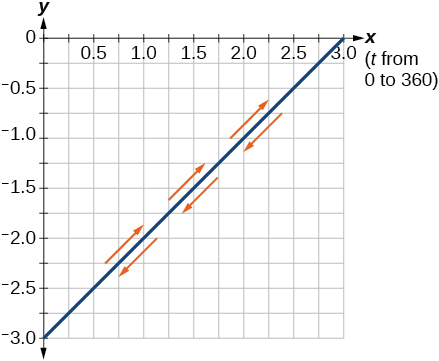$\left\{\begin{array}{l}x\left(t\right)=\mathrm{sec}\text{\hspace{0.17em}}t\\ y\left(t\right)=\mathrm{tan}\text{\hspace{0.17em}}t\end{array}$

$\left\{\begin{array}{l}x\left(t\right)=\mathrm{sec}\text{\hspace{0.17em}}t\\ y\left(t\right)={\mathrm{tan}}^{2}t\end{array}$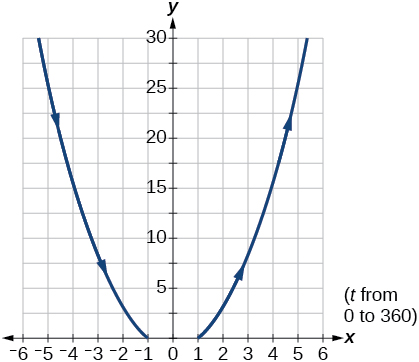$\left\{\begin{array}{l}x\left(t\right)=\frac{1}{{e}^{2t}}\\ y\left(t\right)={e}^{-\text{\hspace{0.17em}}t}\end{array}$

For the following exercises, graph the equation and include the orientation. Then, write the Cartesian equation.

$\left\{\begin{array}{l}x\left(t\right)=t-1\hfill \\ y\left(t\right)=-{t}^{2}\hfill \end{array}$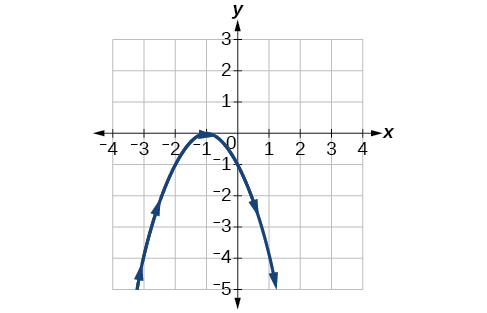$\left\{\begin{array}{l}x\left(t\right)={t}^{3}\hfill \\ y\left(t\right)=t+3\hfill \end{array}$

$\left\{\begin{array}{l}x\left(t\right)=2\mathrm{cos}\text{\hspace{0.17em}}t\\ y\left(t\right)=-\mathrm{sin}\text{\hspace{0.17em}}t\end{array}$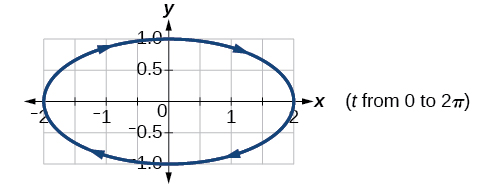$\left\{\begin{array}{l}x\left(t\right)=7\mathrm{cos}\text{\hspace{0.17em}}t\\ y\left(t\right)=7\mathrm{sin}\text{\hspace{0.17em}}t\end{array}$

$\left\{\begin{array}{l}x\left(t\right)={e}^{2t}\\ y\left(t\right)=-{e}^{\text{\hspace{0.17em}}t}\end{array}$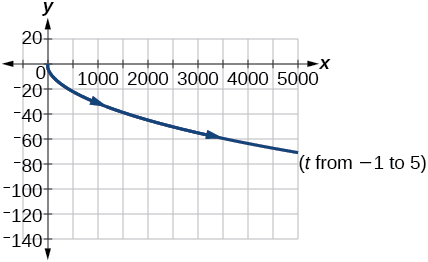For the following exercises, graph the equation and include the orientation.

$x={t}^{2},\text{\hspace{0.17em}}y\text{\hspace{0.17em}}=\text{\hspace{0.17em}}3t,\text{\hspace{0.17em}}0\le t\le 5$

$x=2t,\text{\hspace{0.17em}}y\text{\hspace{0.17em}}=\text{\hspace{0.17em}}\text{\hspace{0.17em}}{t}^{2},\text{\hspace{0.17em}}-5\le t\le 5$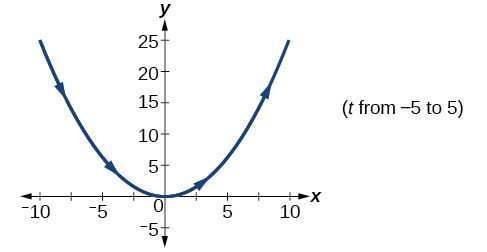$x=t,\text{\hspace{0.17em}}y=\sqrt{25-{t}^{2}},\text{\hspace{0.17em}}0

$x\left(t\right)=-t,y\left(t\right)=\sqrt{t},\text{\hspace{0.17em}}t\ge 0$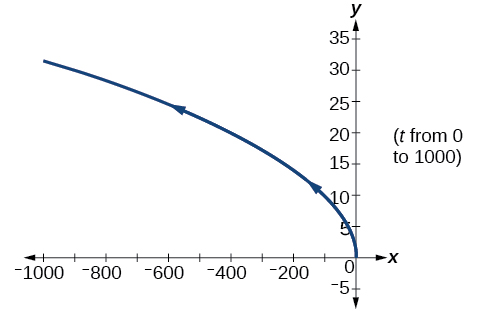$x=-2\mathrm{cos}\text{\hspace{0.17em}}t,\text{\hspace{0.17em}}y=6\text{\hspace{0.17em}}\mathrm{sin}\text{\hspace{0.17em}}t,\text{\hspace{0.17em}}0\le t\le \pi$

$x=-\mathrm{sec}\text{\hspace{0.17em}}t,\text{\hspace{0.17em}}y=\mathrm{tan}\text{\hspace{0.17em}}t,\text{\hspace{0.17em}}-\frac{\text{\hspace{0.17em}}\pi }{2}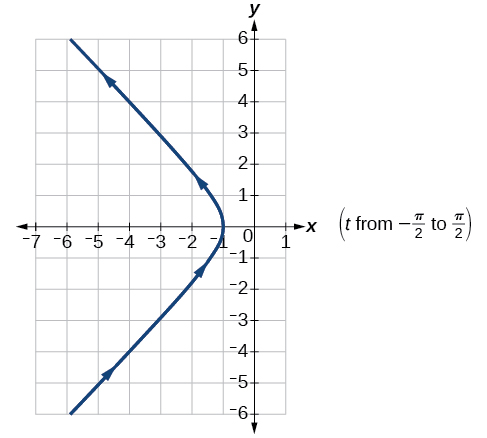For the following exercises, use the parametric equations for integers a and b :

$\begin{array}{l}x\left(t\right)=a\mathrm{cos}\left(\left(a+b\right)t\right)\\ y\left(t\right)=a\mathrm{cos}\left(\left(a-b\right)t\right)\end{array}$

Graph on the domain $\text{\hspace{0.17em}}\left[-\pi ,0\right],\text{\hspace{0.17em}}$ where $\text{\hspace{0.17em}}a=2\text{\hspace{0.17em}}$ and $\text{\hspace{0.17em}}b=1,\text{\hspace{0.17em}}$ and include the orientation.

Graph on the domain $\text{\hspace{0.17em}}\left[-\pi ,0\right],\text{\hspace{0.17em}}$ where $\text{\hspace{0.17em}}a=3\text{\hspace{0.17em}}$ and $\text{\hspace{0.17em}}b=2$ , and include the orientation.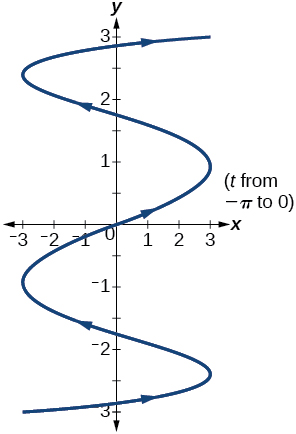Graph on the domain $\text{\hspace{0.17em}}\left[-\pi ,0\right],\text{\hspace{0.17em}}$ where $\text{\hspace{0.17em}}a=4\text{\hspace{0.17em}}$ and $\text{\hspace{0.17em}}b=3$ , and include the orientation.

find the equation of the line if m=3, and b=-2
graph the following linear equation using intercepts method. 2x+y=4
Ashley
how
Wargod
what?
John
ok, one moment
UriEl
how do I post your graph for you?
UriEl
it won't let me send an image?
UriEl
also for the first one... y=mx+b so.... y=3x-2
UriEl
y=mx+b you were already given the 'm' and 'b'. so.. y=3x-2
Tommy
"7"has an open circle and "10"has a filled in circle who can I have a set builder notation
x=-b+_Гb2-(4ac) ______________ 2a
I've run into this: x = r*cos(angle1 + angle2) Which expands to: x = r(cos(angle1)*cos(angle2) - sin(angle1)*sin(angle2)) The r value confuses me here, because distributing it makes: (r*cos(angle2))(cos(angle1) - (r*sin(angle2))(sin(angle1)) How does this make sense? Why does the r distribute once
so good
abdikarin
this is an identity when 2 adding two angles within a cosine. it's called the cosine sum formula. there is also a different formula when cosine has an angle minus another angle it's called the sum and difference formulas and they are under any list of trig identities
strategies to form the general term
carlmark
consider r(a+b) = ra + rb. The a and b are the trig identity.
Mike
How can you tell what type of parent function a graph is ?
generally by how the graph looks and understanding what the base parent functions look like and perform on a graph
William
if you have a graphed line, you can have an idea by how the directions of the line turns, i.e. negative, positive, zero
William
y=x will obviously be a straight line with a zero slope
William
y=x^2 will have a parabolic line opening to positive infinity on both sides of the y axis vice versa with y=-x^2 you'll have both ends of the parabolic line pointing downward heading to negative infinity on both sides of the y axis
William
y=x will be a straight line, but it will have a slope of one. Remember, if y=1 then x=1, so for every unit you rise you move over positively one unit. To get a straight line with a slope of 0, set y=1 or any integer.
Aaron
yes, correction on my end, I meant slope of 1 instead of slope of 0
William
what is f(x)=
I don't understand
Joe
Typically a function 'f' will take 'x' as input, and produce 'y' as output. As 'f(x)=y'. According to Google, "The range of a function is the complete set of all possible resulting values of the dependent variable (y, usually), after we have substituted the domain."
Thomas
Sorry, I don't know where the "Â"s came from. They shouldn't be there. Just ignore them. :-)
Thomas
Darius
Thanks.
Thomas
Â
Thomas
It is the Â that should not be there. It doesn't seem to show if encloses in quotation marks. "Â" or 'Â' ... Â
Thomas
Now it shows, go figure?
Thomas
what is this?
i do not understand anything
unknown
lol...it gets better
Darius
I've been struggling so much through all of this. my final is in four weeks 😭
Tiffany
this book is an excellent resource! have you guys ever looked at the online tutoring? there's one that is called "That Tutor Guy" and he goes over a lot of the concepts
Darius
thank you I have heard of him. I should check him out.
Tiffany
is there any question in particular?
Joe
I have always struggled with math. I get lost really easy, if you have any advice for that, it would help tremendously.
Tiffany
Sure, are you in high school or college?
Darius
Hi, apologies for the delayed response. I'm in college.
Tiffany
how to solve polynomial using a calculator
So a horizontal compression by factor of 1/2 is the same as a horizontal stretch by a factor of 2, right?
The center is at (3,4) a focus is at (3,-1), and the lenght of the major axis is 26
The center is at (3,4) a focus is at (3,-1) and the lenght of the major axis is 26 what will be the answer?
Rima
I done know
Joe
What kind of answer is that😑?
Rima
I had just woken up when i got this message
Joe
Rima
i have a question.
Abdul
how do you find the real and complex roots of a polynomial?
Abdul
@abdul with delta maybe which is b(square)-4ac=result then the 1st root -b-radical delta over 2a and the 2nd root -b+radical delta over 2a. I am not sure if this was your question but check it up
Nare
This is the actual question: Find all roots(real and complex) of the polynomial f(x)=6x^3 + x^2 - 4x + 1
Abdul
@Nare please let me know if you can solve it.
Abdul
I have a question
juweeriya
hello guys I'm new here? will you happy with me
mustapha
The average annual population increase of a pack of wolves is 25.
how do you find the period of a sine graph
Period =2π if there is a coefficient (b), just divide the coefficient by 2π to get the new period
Am
if not then how would I find it from a graph
Imani
by looking at the graph, find the distance between two consecutive maximum points (the highest points of the wave). so if the top of one wave is at point A (1,2) and the next top of the wave is at point B (6,2), then the period is 5, the difference of the x-coordinates.
Am
you could also do it with two consecutive minimum points or x-intercepts
Am
I will try that thank u
Imani
Case of Equilateral Hyperbola
ok
Zander
ok
Shella
f(x)=4x+2, find f(3)
Benetta
f(3)=4(3)+2 f(3)=14
lamoussa
14
Vedant
pre calc teacher: "Plug in Plug in...smell's good" f(x)=14
Devante
8x=40
Chris
Explain why log a x is not defined for a < 0
the sum of any two linear polynomial is whatByByByByByBy